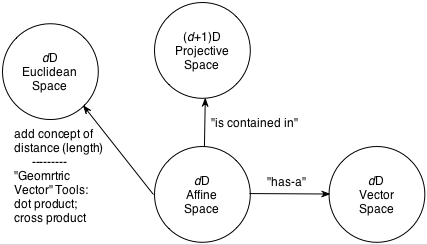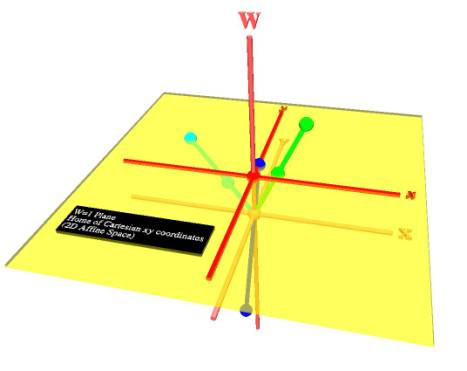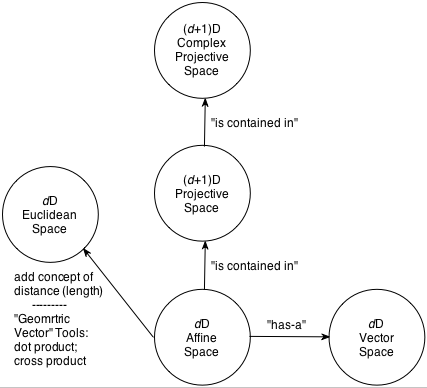# Understanding Geometry:

## The Abstract Spaces in Which Points and Vectors Live

### Vector Spaces

Definition: A d-dimensional Vector Space consists of (i) a set of vectors, and (ii) two operations: addition and scalar multiplication. The vector space is closed under these two operations. There is a distinguished member of the set called the zero vector, 0, with the properties:

∀ real numbers, a: a·0 = 0
∀ vectors, v, in the vector space: 0 + v = v + 0 = v

### Affine Spaces

Definition: A d-dimensional Affine Space consists of (i) a set of points, (ii) an associated d-dimensional vector space, and (iii) two operations: (iii.a) subtraction of two points in the set (which yields a vector in the associated vector space), and (iii.b) addition of a point in the affine space to a vector in the associated vector space (which yields another point in the affine space).

Unlike a vector space which has the distinguished element 0, there is no distinguished point of an affine space.

1. These two definitions define an abstract algebra for abstract points and vectors. Our interest will be in what we will call geometric points and vectors, specifically points and vectors in 2D (d=2) and 3D (d=3) space.
• Since they are first and foremost members of these two spaces, all of their formal properties – including the set of valid operations we can perform on them – derive from these two definitions. Note in particular that the definitions dictate that you can only add and scale vectors, and that you can only subtract two points and add a point and a vector. This seems extremely limited – you cannot scale a point like you can scale a vector, for example – but we will soon discover a few higher level and very familiar operations that can be proven to be valid and consistent with the restrictions embodied in the two definitions.
• Geometric points are characterized as a position in space. While they are typically referenced via coordinates expressed in some reference coordinate system, it is important to recognize that a point exists independent of any coordinate system
• Geometric vectors are characterized as a direction and a length. Vectors have no position.
2. An additional property of interest related to geometric points and vectors relates to measuring lengths and will allow us to characterize such quantities as the distance between points (and hence the sizes of objects) and lengths of vectors. But notice that neither the vector space definition nor the affine space definition includes any notion of distance or size. We therefore need another formalism – another space. Our geometric points and vectors live in a Euclidean Space as described next.

### Euclidean Spaces

Definition: A d-dimensional Euclidean Space is a d-dimensional Affine Space with the additional concept of distance or length.

Two additional extremely important vector operations (whose definitions involve lengths) can be defined for Euclidean Spaces:

1. The dot product (also called the inner product) of two vectors in the vector space: The dot product produces a scalar (i.e., a real number).
2. The cross product: In 3D vector spaces, the cross product of two vectors in the vector space produces another vector in the vector space.

### Projective SpacesDefinition: A (d+1)-dimensional projective space is a space in which the points of a d-dimensional affine space are embedded. We denote the extra coordinate dimension as w and say that the entire set of d-dimensional affine points lies in the w=1 plane of the projective space. All projective space points on the line from the projective space origin through an affine point on the w=1 plane are said to be projectively equivalent to one another (and hence to the affine space point). In three-dimensional affine space, for example, the affine space point R=(x,y,z) is projectively equivalent to all points RP=(wx, wy, wz, w) for w≠0.

Points in projective space will be of interest to us in two contexts:

• When computing the perspective projection of points in a 3D scene, we will use a matrix that generates 4D points that are the projective equivalents of the affine points we seek.
• Rational curves and surfaces (e.g., rational Bezier and rational B-Splines) can be defined using points in projective space whose projectively equivalent affine space points – called "control points" – influence the shape of the curve or surface.

### Complex Projective SpacesDefinition: A (d+1)-dimensional complex projective space is a (d+1)-dimensional projective space in which the coordinates of points are allowed to be complex numbers.

This most general of the spaces we will study is important in part because many fundamental theorems of Geometric Modeling (e.g.,Bezout’s Theorem related to curve and surface intersections) are proven in complex projective space.